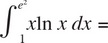# AP Calculus BC Practice Test 6

### Test Information10 questions20 minutes

Calculator Disallowed

1. What is the slope of the line tangent to the curve x2 + 2xy + 3y2 = 2 when y = 1?

2.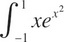dx =

3. If, for t > 0, x = t2 and y = cos(t2), then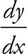=

4. The function f(x) = 4x3 – 8x2 + 1 on the interval [-1, 1] has an absolute minimum at x =

5.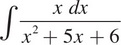6.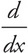(x2 sin2 x) =

7. The line normal to the curve y =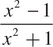at x = 2 has slope

8.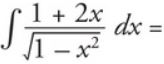9.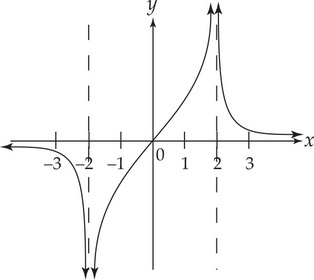The graph of y = f(x) is shown above. Which of the following could be the graph of y = f′(x)?

10.